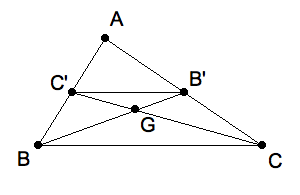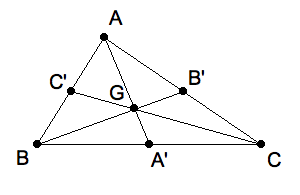This lesson contrasts two methods of proving geometrical theorems and motivate the main theorem of this course.Medians meet at their nether trisectors. (Click image to download centroid.seg for KSEG.)

### Proof in the style of Euclid

Let $G$ be the point where medians $B B'$ and $C C'$ of $\triangle ABC$ intersect. We shall show that $G$ trisects the two medians in the sense that $BG:GB' = 2:1$ and $CG:GC' = 2:1$. This means that any two medians meet at their point two-thirds of the way from the vertex to the midpoint of the opposite side. So all three do.

Prior to the place in Euclid’s Elements are theorems about similar triangles and about angles made by transversals of two parallel lines. The ones we’ll use here are

• simAAA Two triangles are similar if and only if their angles are pairwise equal.

• simSAS Two triangles are simlar if and only if two pairs of corresponding sides have the same proportion and the included angles are equal.

• altIA Two lines are parallel if and only if two alternate interior angles they make with a transversal are equal.

• sameSA Two lines are parallel if and only if corresponding angles on the same side of a transversal are equal.

Step 1: Apply simSAS to $\triangle AC'B'$ and $\triangle ABC$ by noting that they have $\angle A$ in common, and the adjacent sides are in the ratio of $1:2$. So the two triangles are similar. That, by definition of similartity, implies that $\angle B'C'A = \angle CBA$ and $C'B':BC = 1:2$.

Step 2: Apply sameSA to the two lines $C'B'$ and $BC$ to the first consequence : $\angle B'C'A = \angle CBA$. Therefore $C'B' \|\| BC$. That in turn, by sameSA, implies that $\angle GC'B' = \angle GCB$ and $\angle C'B'G = \angle CBG$

Step 3: Apply simAAA to the two triangles $\triangle GB'C'$ and $GBC$. Two pairs of their angles have already been shown to be equal. The third pair, $\angle B'GC'$ and $\angle BGC$, are equal because they are "opposite angles". Thus the two triangles are similar.

Step 4: From the second conclusion in Step 1 we know the ratio of to be $2:1$. So $1:2 = GB':GB$ and $1:2 = GC':GC$. So $G$ does "trisect" two of the medians, as predicted.Medians of a triangle are concurrent at the centroid of a triangle.

### Proof in the style of Descartes

Direct observation of a few examples suggests that the medians of a triangle not only meet at the same point, but that this point is two-thirds of the way from the vertex to the midpoint of the opposite side on each median. Call this point the "nether trisector" for short.

Step 1: The nether trisector of the median from vertex $A$ is $G = \frac{1}{3}A + \frac{2}{3}A'$

Step 2: The midpoint of the side opposite $A$ is $A' = \frac{1}{2}B + \frac{1}{2}C$.

Step 3: Substituting and simplifying we get $G = \frac{1}{3}A + \frac{1}{3}B + \frac{1}{3}C$.

Step 4: The expression for $G$ is logically symmetric in all three vertices, i.e. you can’t tell whether you got to Step 4 by starting with vertex $A$ or either $B, C$. So $G$ must be the nether trisector on each median.

## Generalization

One way that mathematics progresses is to generalize a theorem. This entails weakening the hypotheses, and/or strengthening the conclusions. In the above we have already seen how to strengthen the conclusion of the Centroid Theorem as stated at the beginning. Not only are the medians concurrent, we know exactly where on each median the common point is located.

In preparation for the lesson on Ceva’s theorem let’s see how we might weaken the hypotheses. You should use KSEG to follow this discussion. First we restate the theorem in a way that makes it clear what the hypotheses and the conlusions are.

We would not wish to relax the hypotheses that $\triangle ABC$ by omitting it. For the only alternative would be for $(ABC)$ to be collinear, and the notion of "median" would not make much sense.

We might investigate the case that the triangle becomes a more general polygon, for instance a quadrilateral. Or we might consider what happens if the triangle acquires another dimension and becomes a tetrahedron. You might experiment with KSEG to see if you can discover any theorems about "medians". Of course, you need to also generalize the notion of median.

Keeping with a triangle, suppose we relax the need for the points $A', B', C'$ to be midpoints on the opposite side. If we move $A'$ along $(BC)$ the concurrency is destroyed immediately. But if you then move $B'$ carefully, it can be restored. What you have changed is the proportion of segments from $A'$ to $B$ and $C$. We express this ratio as $\frac{A'-B}{A'-C}$. There are three such ratios, and you might never guess what the relationship is between them when concurrency happens.

We pursue these ideas in subsequent lessons.

Corrected 10sep09 by WYS.